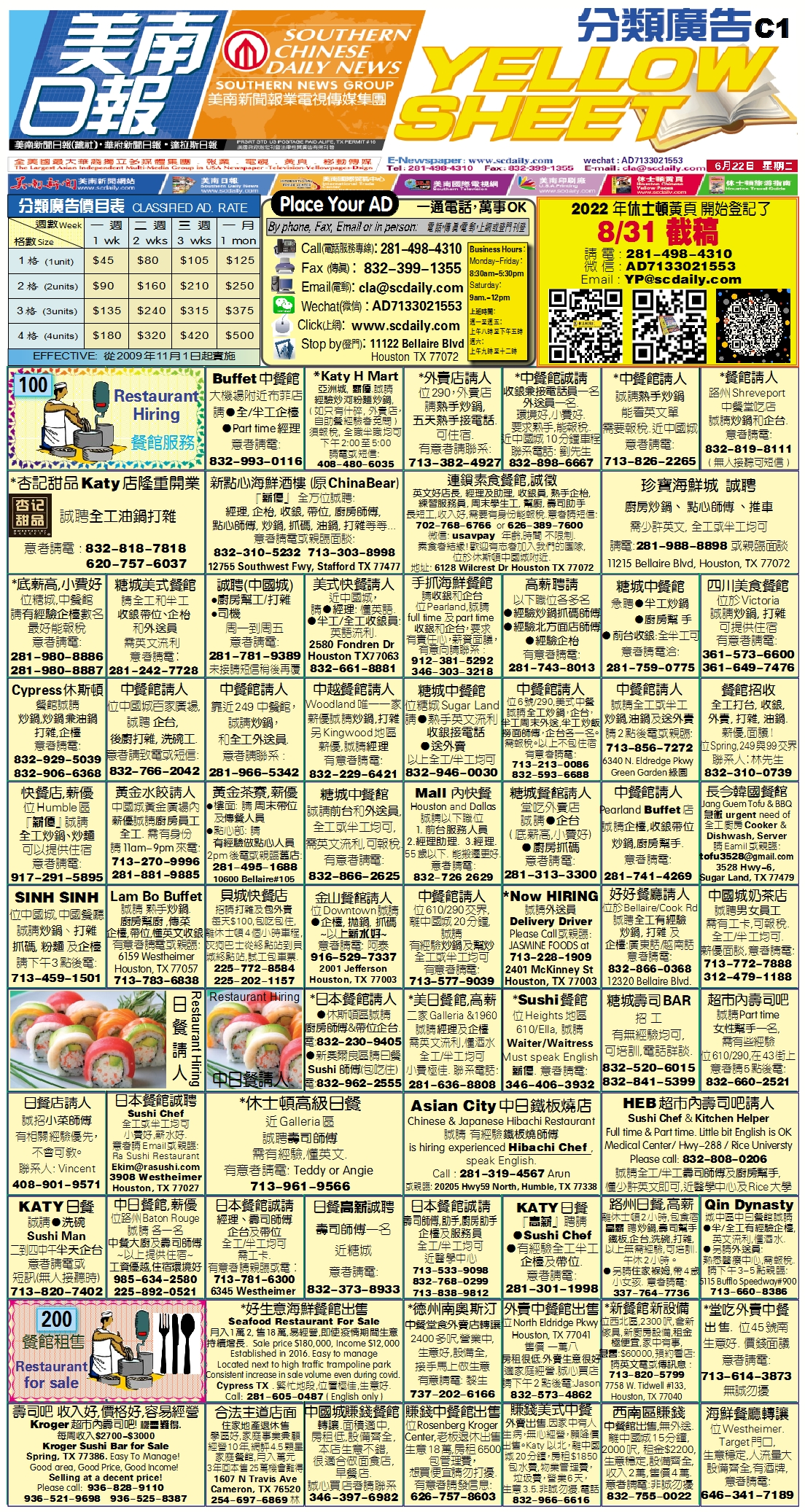210702 Epaper

 A Section B SectionC Section D Section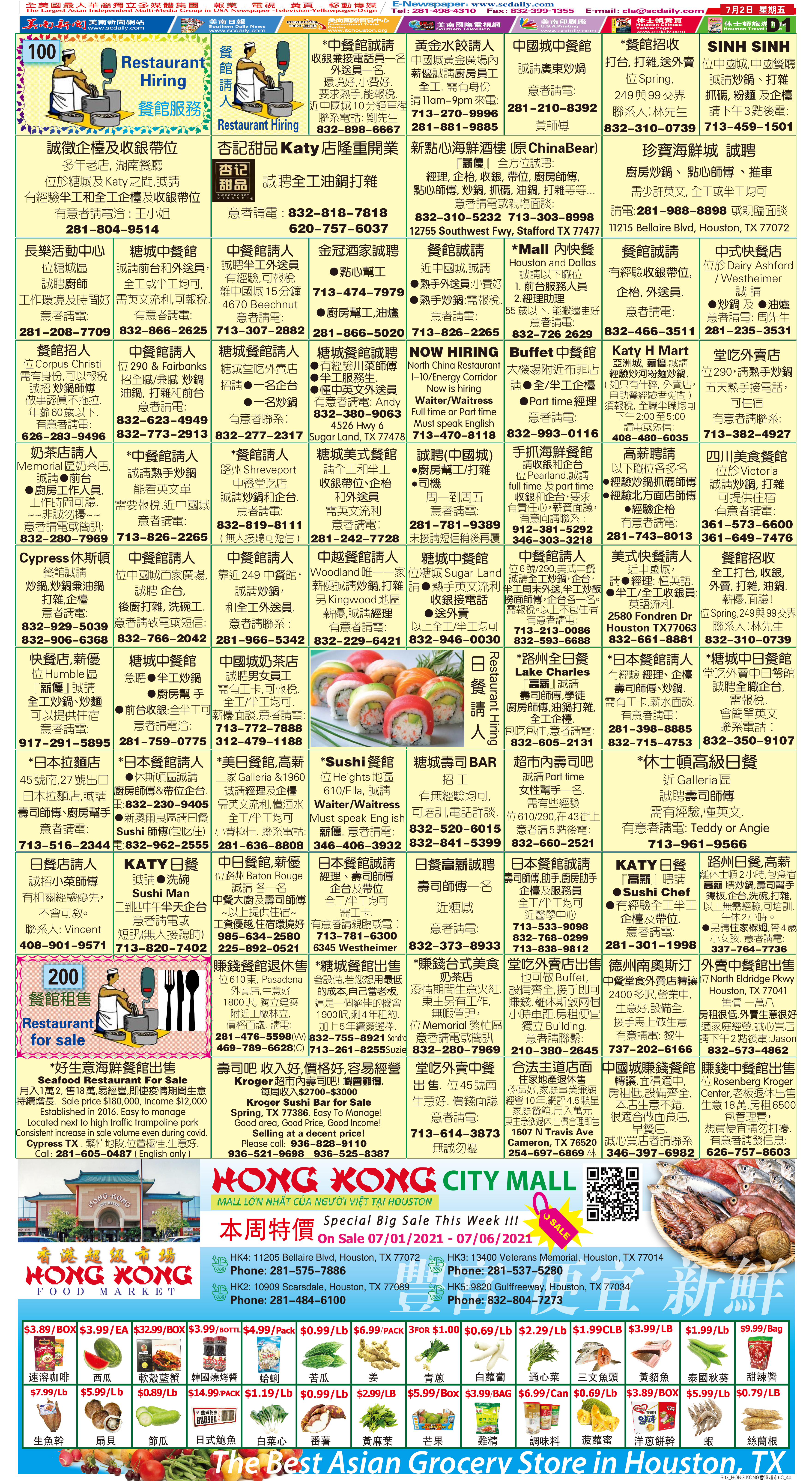210701 Epaper

 A Section B SectionC Section D Section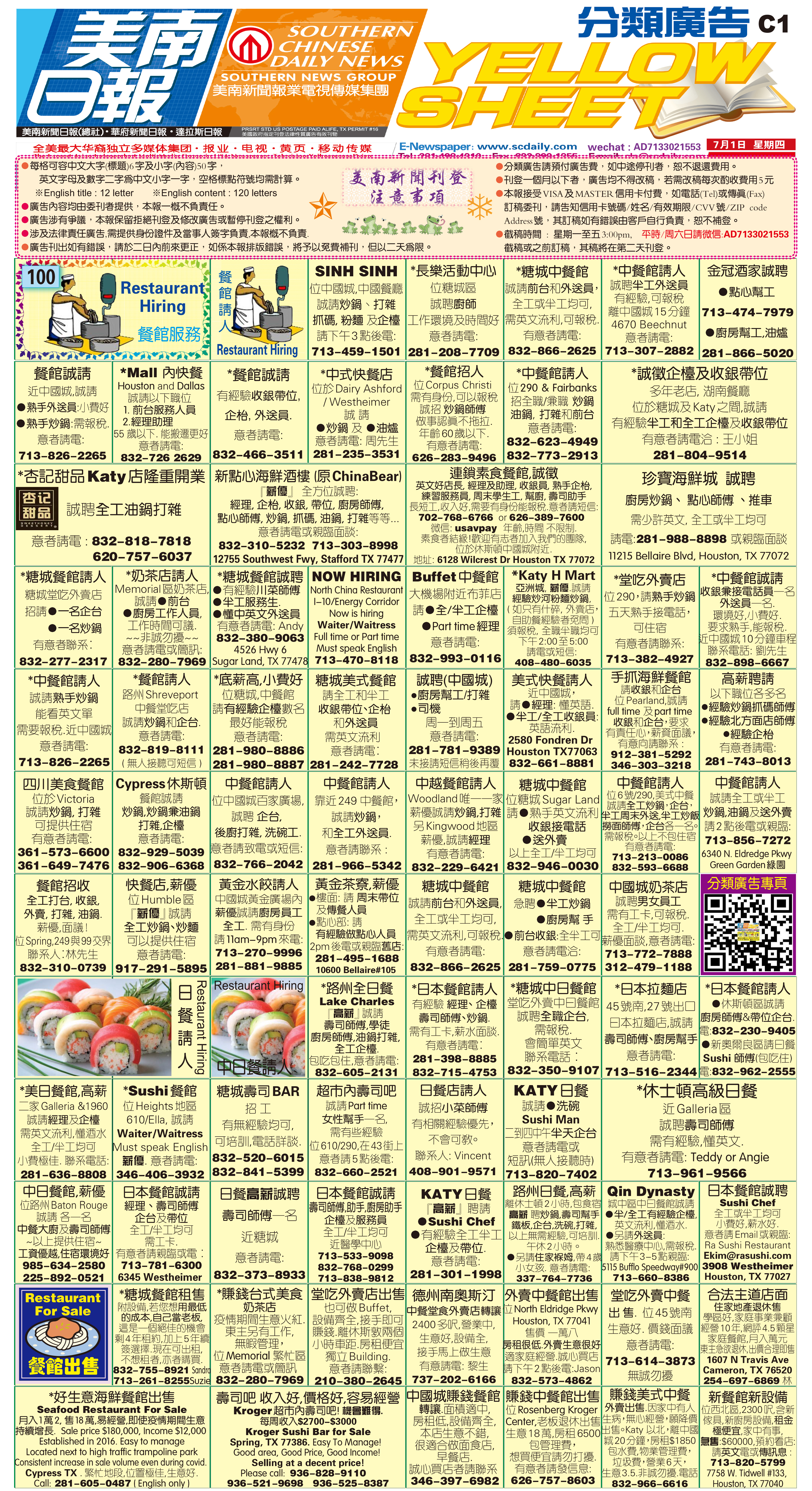210630 Epaper

 A Section B SectionC Section D Section210629 Epaper

 A Section B SectionC Section D Section210628 Epaper

 A Section B SectionC Section D Section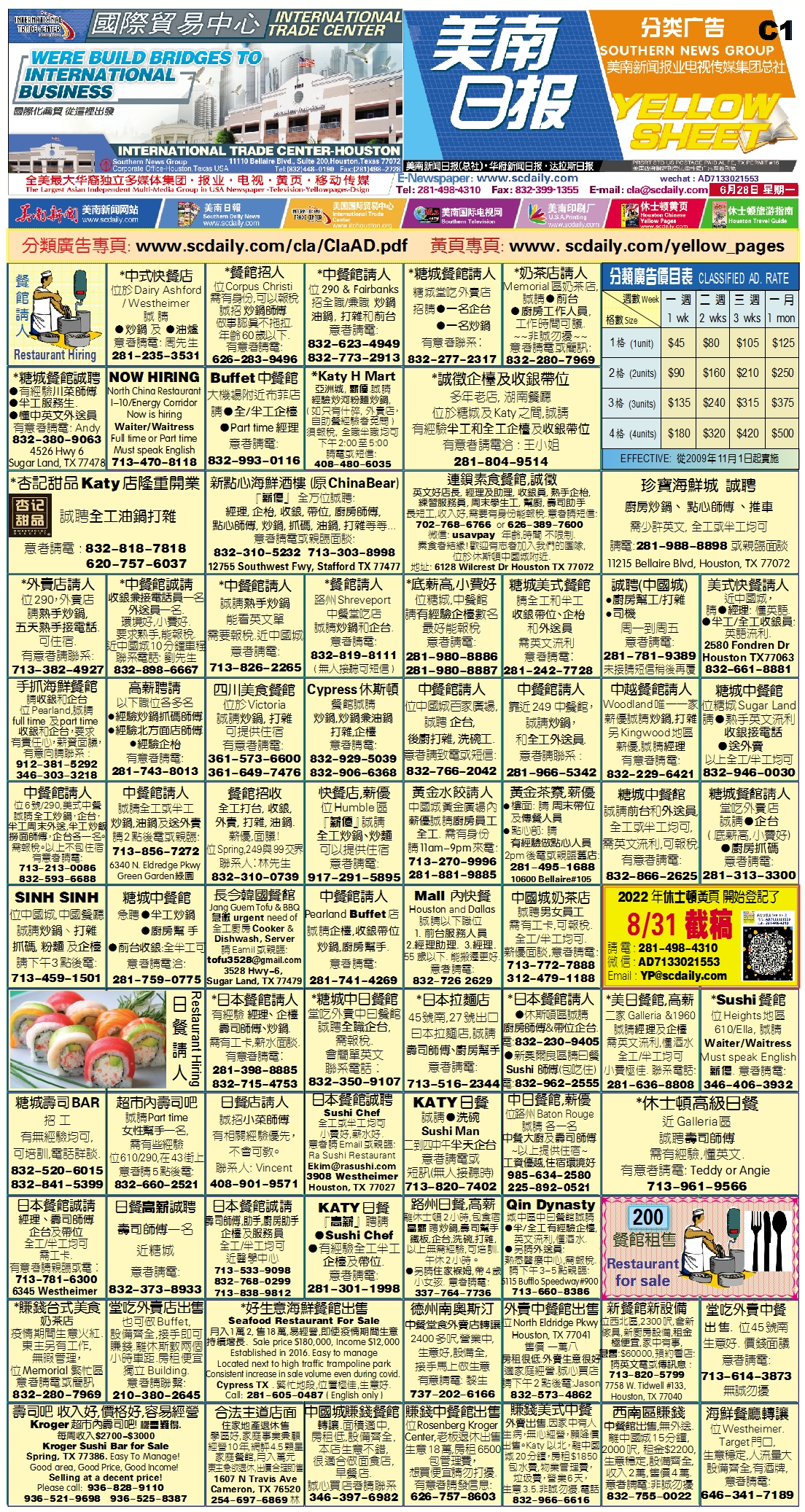210627 Epaper

 A Section B SectionC Section D Section210626 Epaper

 A Section B SectionC Section D Section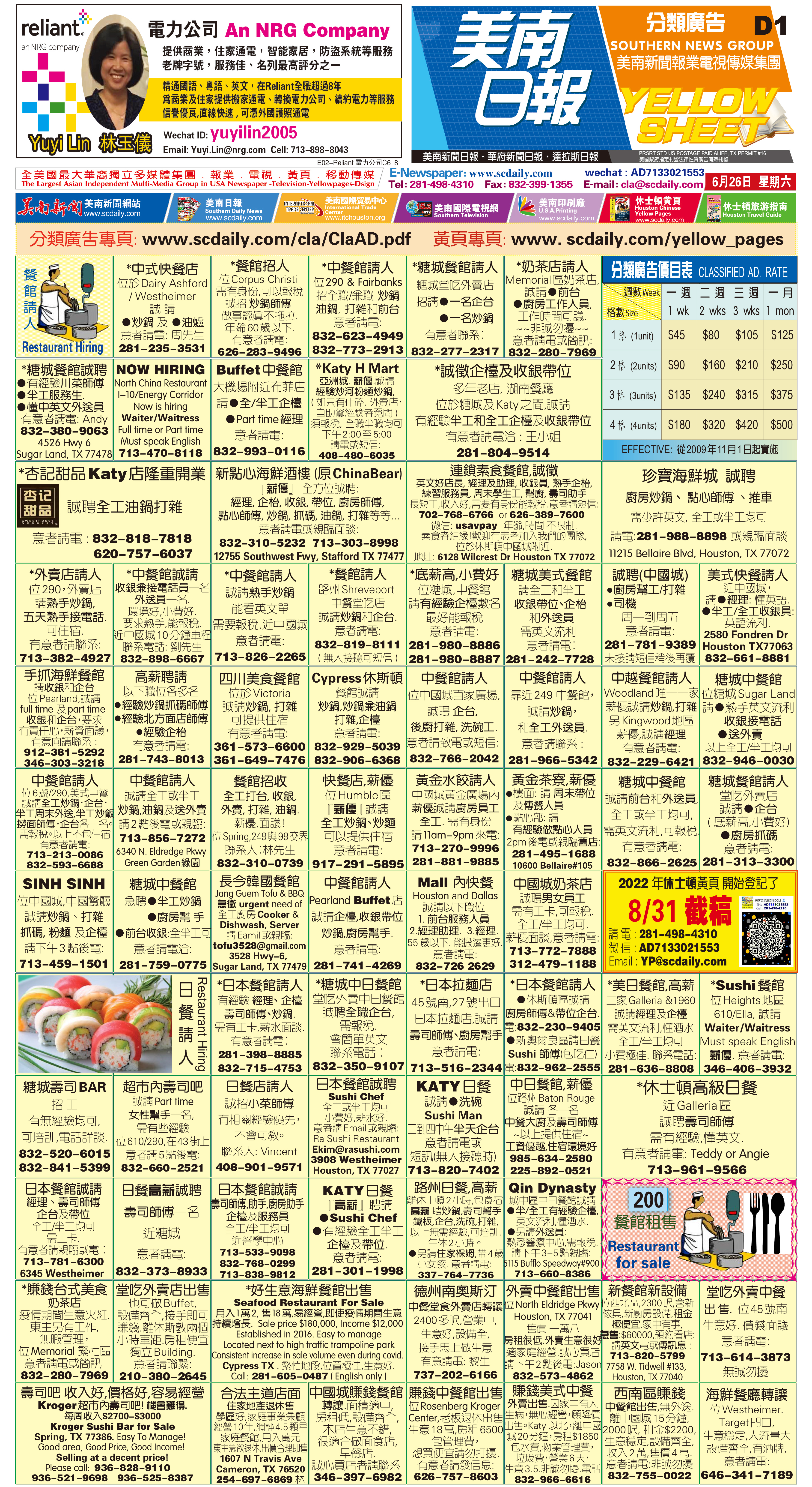210625 Epaper

 A Section B SectionC Section D Section210623 Epaper

 A Section B SectionC Section D Section210624 Epaper

 A Section B SectionC Section D Section210622 Epaper

 A Section B SectionC Section D Section